# ���۱�������������ַ-����������̻��Ǻܿ��׵�

�ҹ���ѡ�˱�Ǯ���͡�����ͳ�����۷ɴ����������˷���ʹ����һϵ�е��²�Ʒ��ȥ����³�����ϣ�ʹ��ҵ������һֱ�����ȶ�����������������������150������Σ����󲿷����ţ��е��Ѿ���ְ�����У����е�ȥ����ش��š�
08高考数学充要条件的判定测试充分条件、必要条件和充要条件是重要的数学概念，主要用来区分命题的条件p和结论q之间的关系.本节主要是通过不同的知识点来剖析充分必要条件的意义，让考生能准确判定给定的两个命题的充要关系.●难点磁场()已知关于x的实系数二次方程x2+ax+b=0有两个实数根α、β，证明：|α|<2且|β|<2是2|a|<4+b且|b|<4的充要条件.●案例探究［例1］已知p：|1－|≤2,q:x2－2x+1－m2≤0(m>0),若⌐p
• 1.()函数f(x)=x|x+a|+b是奇函数的充要条件是(　　 )

A.ab=0　　　　　　　　　　　　 B.a+b=0　　　　　　　　　　　 C.a=b　　　　　　　　　 D.a2+b2=0

• 2.()“a=1”是函数y=cos2ax－sin2ax的最小正周期为“π”的(　　 )

A.充分不必要条件　　　　　　　　　　　　　　　　　　　　　　　　 B.必要不充分条件

C.充要条件　　　　　　　　　　　　　　　　　　　　　　　　　　 D.既非充分条件也不是必要条件

• 3.()a=3是直线ax+2y+3a=0和直线3x+(a－1)y=a－7平行且不重合的_________.

• 4.()命题A：两曲线F(x,y)=0和G(x,y)=0相交于点P(x0,y0),命题B：曲线F(x,y)+λG(x,y)=0(λ为常数)过点P(x0,y0),则AB的__________条件.

• 5.()设αβ是方程x2ax+b=0的两个实根，试分析a>2且b>1是两根αβ均大于1的什么条件？

• 6.()已知数列{an}、{bn}满足：bn=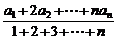,求证：数列{an}成等差数列的充要条件是数列{bn}也是等差数列.

• 7.()已知抛物线Cy=－x2+mx－1和点A(3，0)，B(0，3)，求抛物线C与线段AB有两个不同交点的充要条件.

• 8.()p:－2<m<0,0<n<1;q:关于x的方程x2+mx+n=0有2个小于1的正根，试分析pq的什么条件.(充要条件)

08高考数学充要条件的判定测试充分条件、必要条件和充要条件是重要的数学概念，主要用来区分命题的条件p和结论q之间的关系.本节主要是通过不同的知识点来剖析充分必要条件的意义，让考生能准确判定给定的两个命题的充要关系.●难点磁场()已知关于x的实系数二次方程x2+ax+b=0有两个实数根α、β，证明：|α|<2且|β|<2是2|a|<4+b且|b|<4的充要条件.●案例探究［例1］已知p：|1－|≤2,q:x2－2x+1－m2≤0(m>0),若⌐p参考答案

f(x)=x2+ax+b,则f(x)的图象是开口向上的抛物线.

(2)必要性：

∴方程f(x)=0的两根αβ同在(－2，2)内或无实根.

αβ是方程f(x)=0的实根，

αβ同在(－2，2)内，即|α|＜2且|β|＜2.

=－(x|x+a|+b)=－f(x).

a2+b2=0是f(x)为奇函数的充分条件，又若f(x)=x|x+a|+b是奇函数，即f(－x)=

(－x)|(－x)+a|+b=－f(x),则必有a=b=0,即a2+b2=0.

a2+b2=0是f(x)为奇函数的必要条件.

2.解析：若a=1,则y=cos2x－sin2x=cos2x,此时y的最小正周期为π.故a=1是充分条件，反过来，由y=cos2ax－sin2ax=cos2ax.故函数y的最小正周期为π,则a=±1,故a=1不是必要条件.

4.解析：若P(x0,y0)是F(x,y)=0和G(x,y)=0的交点，则F(x0,y0)+λG(x0,y0)=0，即F(x,y)+λG(x,y)=0，过P(x0,y0);反之不成立.

(1)由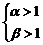，得a=α+β>2,b=αβ>1,∴q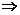p

(2)为证明p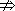q,可以举出反例：取α=4,β=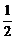,它满足a=α+β=4+>2,b=αβ=4×=2>1,但q不成立.

6.证明：①必要性：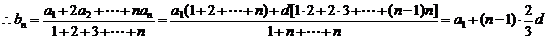从而bn+1bn=a1+n.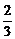da1－(n－1)d=d为常数.

故{bn}是等差数列，公差为d.

②充分性:

bn(1+2+…+n)=a1+2a2+…+nan

bn1(1+2+…+n－1)=a1+2a2+…+(n－1)an

①－②得：nan=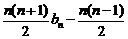bn1

an=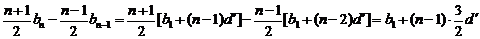,从而得an+1an=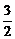d′为常数，故{an}是等差数列.

7.解：①必要性：

f(x)=x2－(m+1)x+4,则有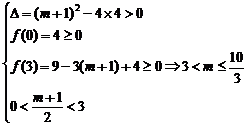②充分性：

x1=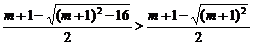>0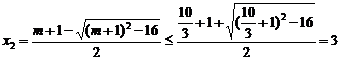∴方程x2－(m+1)x+4=0有两个不等的实根x1,x2,且0＜x1x2≤3,方程组*有两组不同的实数解.

8.解：若关于x的方程x2+mx+n=0有2个小于1的正根，设为x1,x2.